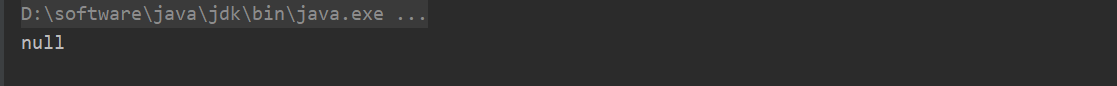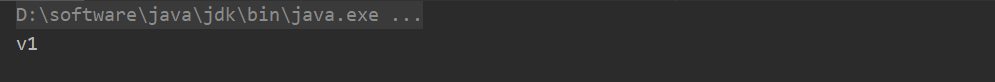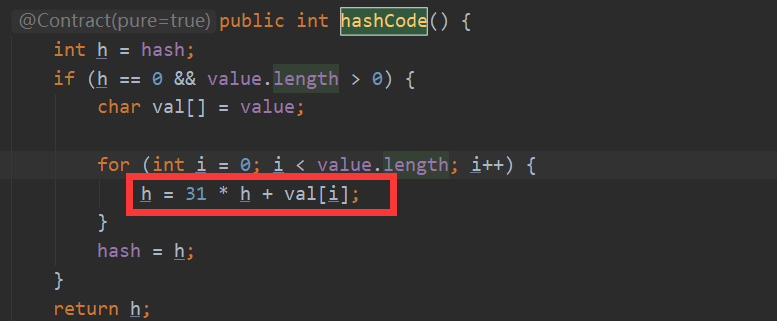# Java入门系列之hashCode和equals

## equals

1、自反性：对于非空的对象x，x.equals(x)必须返回true.

2、对称性：对于非空的对象x和y，若x.equals(y)等于true时，那么y.equals(x)也必须返回true.

3、传递性：对于非空的对象x、y和z，如果x.equals(y)和y.equals(z)等于true时，那么x.equals(z)也必须返回true

4、一致性：对于非空的对象x和y，如果利用equals判断对象的信息没有被修改时，无论调用多少次，那么x.equals(y)要么为true，要么为false

5、对于非空的对象x，x.equals(null)必须返回false

1、使用“==”判断两个对象是否引用相同

2、使用instanceof操作符来检查参数类型是否相同

3、若类型相同，则将参数转换为正确的类型

4、比较对象中每个值是否都相等，若全部相等则返回true，否则为false

    public boolean equals(Object anObject) {
// 判断对象引用是否相等，相等直接返回
if (this == anObject) {
return true;
}
//判断对象参数类型是否正确
if (anObject instanceof String) {

//若参数类型相同，则转换为对应的参数类型
String anotherString = (String)anObject;
int n = value.length;

//比较参数对象中的所有值是否相等
if (n == anotherString.value.length) {
char v1[] = value;
char v2[] = anotherString.value;
int i = 0;
while (n-- != 0) {
if (v1[i] != v2[i])
return false;
i++;
}
return true;
}
}
return false;
}

## hashCode

public class Person {
int age;
String name;

public Person(int age, String name) {
this.age = age;
this.name = name;
}

@Override
public boolean equals(Object obj) {
if (this == obj) {
return true;
}

if (obj instanceof Person) {
Person p = (Person) obj;
return (this.age == p.age && this.name == p.name);
}

return  false;
}
}

        Person p1 = new Person(12, "Jeffcky");
Person p2 = new Person(12, "Jeffcky");

Hashtable hashtable = new Hashtable();
hashtable.put(p1, "v1");

System.out.println(hashtable.get(p2));@Override
public int hashCode() {
return (31 * Integer.valueOf(this.age).hashCode() + name.hashCode());
}上图标记出的就是计算字符串的hashCode核心即散列函数，从上看出通过字符串中每一个字符的ASCII码来计算，同时我们也可再拓展下看源码数值类型的hashCode就是其本身。上述计算方式最终我们数学进行归纳出计算方法为：

s*31^(n-1) + s*31^(n-2) + ... + s[n-1]

65*31^(2-1) + 67*31^(2-2) = 2082

## 总结

posted @ 2019-09-29 14:36  Jeffcky  阅读(718)  评论(1编辑  收藏  举报